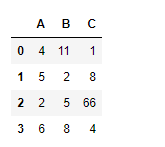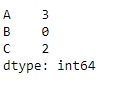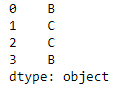GFG App
Open AppBrowser
Continue

# Python | Pandas dataframe.idxmax()

Python is a great language for doing data analysis, primarily because of the fantastic ecosystem of data-centric python packages. Pandas is one of those packages and makes importing and analyzing data much easier.

Pandas` dataframe.idxmax()` function returns index of first occurrence of maximum over requested axis. While finding the index of the maximum value across any index, all NA/null values are excluded.

Syntax: DataFrame.idxmax(axis=0, skipna=True)

Parameters :
axis : 0 or ‘index’ for row-wise, 1 or ‘columns’ for column-wise
skipna : Exclude NA/null values. If an entire row/column is NA, the result will be NA

Returns : idxmax : Series

Example #1: Use `idxmax()` function to function to find the index of the maximum value along the index axis.

 `# importing pandas as pd ` `import` `pandas as pd ` ` `  `# Creating the dataframe  ` `df ``=` `pd.DataFrame({``"A"``:[``4``, ``5``, ``2``, ``6``],  ` `                   ``"B"``:[``11``, ``2``, ``5``, ``8``], ` `                   ``"C"``:[``1``, ``8``, ``66``, ``4``]}) ` ` `  `# Print the dataframe ` `df `Now apply the `idxmax()` function along the index axis.

 `# applying idxmax() function. ` `df.idxmax(axis ``=` `0``) `

Output :If we look at the values in the dataframe, we can verify the result returned by the function. The function returned a pandas series object containing the index of maximum value in each column.

Example #2: Use `idxmax()` function to find the index of the maximum value along the column axis. The dataframe contains `NA` values.

 `# importing pandas as pd ` `import` `pandas as pd ` ` `  `# Creating the dataframe  ` `df ``=` `pd.DataFrame({``"A"``:[``4``, ``5``, ``2``, ``None``], ` `                   ``"B"``:[``11``, ``2``, ``None``, ``8``],  ` `                   ``"C"``:[``1``, ``8``, ``66``, ``4``]}) ` ` `  `# Skipna = True will skip all the Na values ` `# find maximum along column axis ` `df.idxmax(axis ``=` `1``, skipna ``=` `True``) `

Output :The output is a pandas series containing the column label for each row which has the maximum value.

My Personal Notes arrow_drop_up